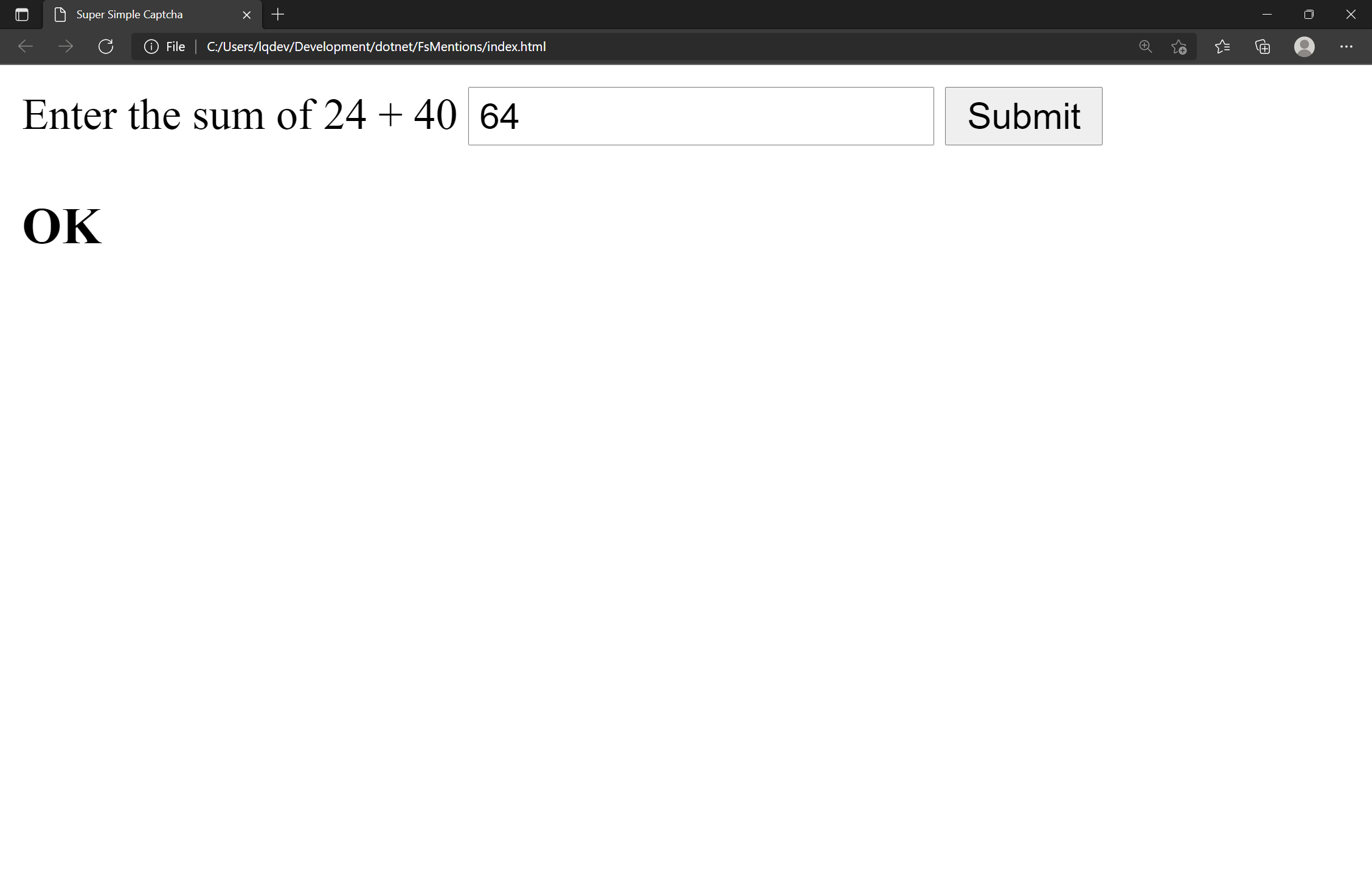## Introduction

While it's great that you can mention and comment on other people's content from your site, like comments, writing a script to submit spam is relatively simple. Therefore, I want to create some sort of CAPTCHA challenge as an initial form of validation to prevent spam. At the same time, I don't want to have any external dependencies. In this post, I'll show how I went about implementing a captcha-like solution with zero dependencies to prevent spam submissions.

The solution I came up with asks the users to add the day of the month (1-31) to a random number between 1-100. While not entirely foolproof, it's "complex" enough that it's not the same thing every time.

Below is what the implementation looks like.The HTML markup for the webpage looks like the following:

``````<!DOCTYPE html>
<html lang="en">
<meta charset="UTF-8">
<meta http-equiv="X-UA-Compatible" content="IE=edge">
<meta name="viewport" content="width=device-width, initial-scale=1.0">
<script src="main.js" type="text/javascript"></script>
<div>
<span id="query"></span>
<button onclick="displayResult()">Submit</button>
</div>
<h3 id="result"></h3>
</body>
</html>
``````

When the `body` element of the page loads, it invokes the `setQuery` function, which displays the challenge the user is supposed to solve in the `query` span element.

The user then submits their answer via the `answerInput` text input box.

The answer is then checked by invoking the `displayResult` function. The `displayResult` function checks the user's answer against the expected answer. If the answer is correct, the text "OK" is displayed on the page's `result` H3 element. Otherwise, the text "Try again" displays on the webpage.

All of the code that handles this logic is in the `main.js` file.

``````let date = new Date()
let day = date.getDate() // Day of the month
let randomNumber = Math.floor(Math.random() * 100) + 1

let setQuery = () => {
let element = document.getElementById('query');
element.innerText = `Enter the sum of \${day} + \${randomNumber}`;
}

let checkAnswer = () => {
}

let displayResult = () => {
let result = checkAnswer() ? "OK" : "Try again" // Ternary function to check if answer is correct
document.getElementById("result").innerText = result;
}
``````

That's all there is to it!

## Conclusion

In this post, I showed how to implement a dependency-free solution to present a challenge to users submitting comments / webmentions to your site. Although the solution isn't foolproof, it's just complex enough any spammers would have to work a little harder. Happy coding!